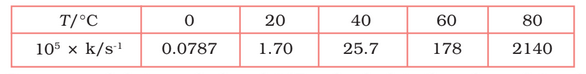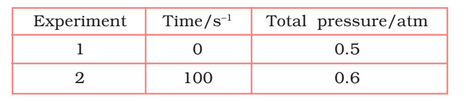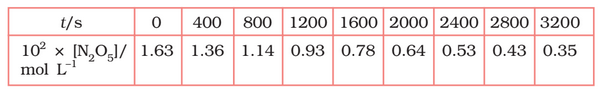## Filters

Sort by :
Clear All
Q

3.20 b) Given the resistances of 1 Ω, 2 Ω, 3 Ω, how will be combine them to get an equivalent resistance of (iii) 6 Ω

series

Question 6. Let ∗ be the binary operation on N given by a ∗ b = L.C.M. of a and b. Find

(v) Which elements of N are invertible for the operation ∗?

test

16. Why is that once a person starts taking alcohol or drugs, it is difficult to get rid of this habit. Discuss it with your teacher.

It's called dependence and addiction. If the use or intake of alcohol is suddenly discontinued it leads to withdrawal symptoms.

Q 15)

Given function is Given function is satisfies for the all real values of x case (i)  k < 0 Hence, function is continuous for all values of x < 0 case (ii)  x = 0 L.H.L at x= 0 R.H.L. at x = 0 L.H.L. = R.H.L. = f(0) Hence, function is continuous at x = 0 case (iii)  k > 0 Hence , function is continuous for all values of x > 0 case (iv) k < 1  Hence , function is...

4.30   The rate of a reaction quadruples when the temperature changes from 293 K to 313 K. Calculate the energy of activation of the reaction assuming that it does not change with temperature.

From the Arrhenius equation, ...................................(i) it is given that  T1= 293 K T2 = 313 K Putting all these values in equation (i) we get, Activation Energy = 52.86 KJ/mol   This is the required activation energy

4.29   The time required for 10% completion of a first order reaction at 298K is equal to that required for its 25% completion at 308K. If the value of A is . Calculate k at 318K and Ea.

We know that, for a first order reaction- Case 1 At temp. = 298 K    = 0.1054/k Case 2 At temp = 308 K       = 2.2877/k' As per the question    K'/K = 2.7296 From Arrhenius equation,      = 76640.096 J /mol      =76.64 KJ/mol   k at 318 K we have , T =318K                  A=  Now  After putting the calue of given variable, we get  on takingantilog we get,  k =...

4.28  The decomposition of A into product has value of k as at 10°Cand energy of activation 60 kJ mol–1. At what temperature would k be ?

The decomposition of A into a product has a value of k as at 10°C and energy of activation 60 kJ mol–1. K1 =  K2 =   =  60 kJ mol–1 K2 =

4.27   The rate constant for the first order decomposition of is given by the following equation:
.

Calculate  for this reaction and at what temperature will its half-period be 256 minutes?

The Arrhenius equation is given by  taking log on both sides,  ....................(i) given equation, .....................(ii) On comparing both equation we get, activation energy  half life ()  = 256 min k = 0.693/256    With the help of equation (ii),            T =                 = 669 (approx)

4.26   The decomposition of hydrocarbon follows the equation  . Calculate

The Arrhenius equation is given by  .................................(i) given equation, ............................(ii) by comparing equation (i) & (ii) we get, A= 4.51011 per sec Activation energy = 28000  (R = 8.314)                              = 232.792 KJ/mol

4.25   Sucrose decomposes in acid solution into glucose and fructose according to the first order rate law, with hours. What fraction of sample of sucrose remains after 8 hours ?

For first order reaction, given that half life = 3 hrs () Therefore k = 0.693/half-life                     = 0.231 per hour Now,                   = antilog (0.8024)                   = 6.3445 (approx) Therefore fraction of sample of sucrose remains after 8 hrs is 0.157

4.24   Consider a certain reaction A   Products with . Calculatethe concentration of A remaining after 100 s if the initial concentration of A is 1.0 mol

Given that, k =  t = 100 s Here the unit of k is in per sec, it means it is a first-order reaction. therefore,  Hence the concentration of rest test sample is 0.135 mol/L

4.23   The rate constant for the decomposition of hydrocarbons is at 546 K. If the energy of activation is 179.9 kJ/mol, what will be the value of pre-exponential factor.

Given that, k =  = 179.9 KJ/mol T(temp) = 546K According to Arrhenius equation, taking log on both sides,                             = (0.3835 - 5) + 17.2082               = 12.5917 Thus A = antilog (12.5917)          A = 3.9  per sec (approx)

4.22   The rate constant for the decomposition of N2O5 at various temperatures
is given below:Draw a graph between ln k and 1/T and calculate the values of A and
. Predict the rate constant at 30° and 50°C.

From the above data, T/ 0 20 40 60 80 T/K 273 293     313 333 353 () 3.66 3.41 3.19 3.0 2.83 0.0787 1.70 25.7 178 2140 -7.147 -4.075 -1.359 -0.577 3.063 Slope of line  =  According to Arrhenius equations, Slope =    12.30 8.314             = 102.27  Again, When T = 30 +273 = 303 K and 1/T =0.0033K    k =  When T = 50  + 273 = 323 K  and 1/T = 3.1  K k = 0.607 per sec

4.21   The following data were obtained during the first order thermal decomposition of  at a constant volume.Calculate the rate of the reaction when total pressure is 0.65 atm.

The thermal decomposition of  is shown here; After t time, the total pressure  =                                                 So,  thus,  for first order reaction,     now putting the values of pressures, when t = 100s       when      = 0.65 - 0.5    = 0.15 atm So,                              = 0.5 - 0.15                             = 0.35 atm Thus, rate of reaction, when the...

4.20   For the decomposition of azoisopropane to hexane and nitrogen at 543K, the following data are obtained.Calculate the rate constant.

Decompostion is represented by equation- After t time, the total pressure  =                                                 So,  thus,  for first order reaction,     now putting the values of pressures, when t =360sec when t = 270sec So,

4.19   A first order reaction takes 40 min for 30% decomposition. Calculate

For the first-order reaction,               (30% already decomposed and remaining is 70%)      therefore half life = 0.693/k                             =                              = 77.7 (approx)

4.18   For a first order reaction, show that time required for 99% completion is twice the time required for the completion of 90% of reaction.

case 1- for 99% complition,             CASE- II for 90% complition,            Hence proved.

4.17   During nuclear explosion, one of the products is with half-life of 28.1 years. If of   was absorbed in the bones of a newly born baby instead of calcium, how much of it will remain after 10 years and 60 years if it is not lost metabolically.

Given, half life = 21.8 years            = 0.693/21.8             and,  by putting the value we get,                            taking antilog on both sides, [R] = antilog(-0.1071)       = 0.781  Thus 0.781  of  will remain after given 10 years of time. Again,  Thus 0.2278  of  will remain after 60 years.

4.16   The rate constant for a first order reaction is . How much time will it take to reduce the initial concentration of the reactant to its 1/16th  value?

We know that, for first order reaction,           (nearly) Hence the time required is

4.15 (6)   The experimental data for decomposition of     in gas phase at 318K are given below:Calculate the half-life period from k and compare it with (ii).

The half life produce =                                    =
Exams
Articles
Questions